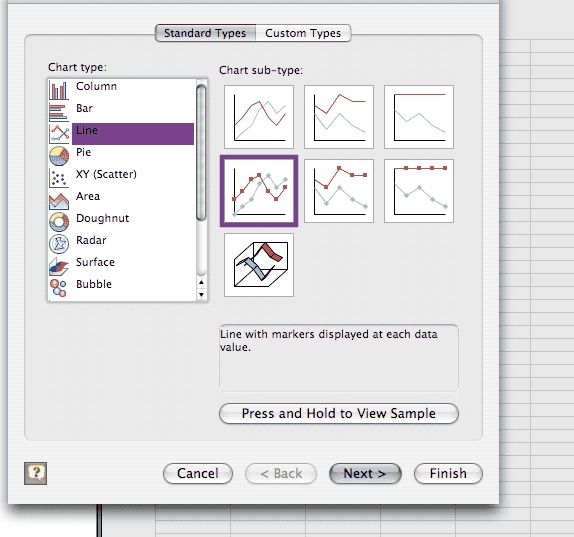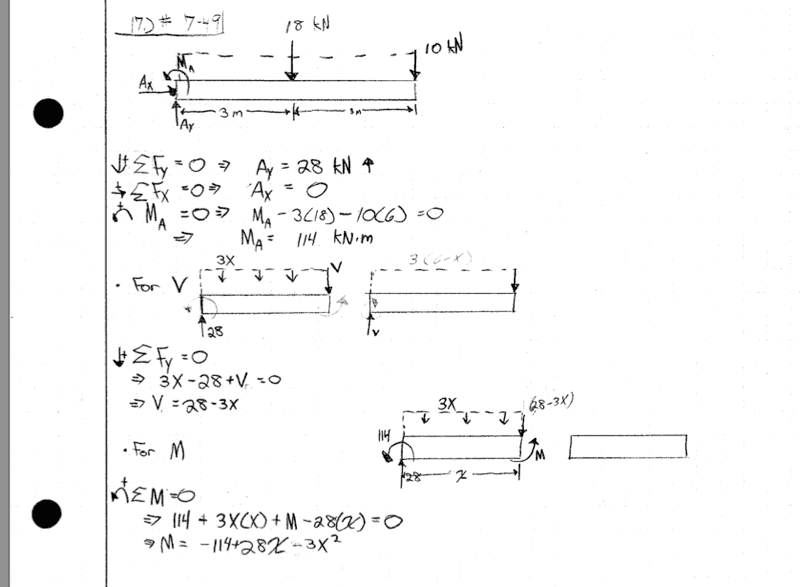# Shear and Bending Moment Diagrams

Shear and Bending Moment Diagrams!!

## Homework Statement## Homework Equations

Newton's 2nd and 3rd

## The Attempt at a Solution

So my main confusion is this:

I found the reaction at A to be 19 kN

If I make a vertical cut through some arbitrary point along the beam and and do some analysis I get:

From the left portion:
$$\downarrow +\sum F_y=0\Rightarrow V-19+3x=0\Rightarrow V=19-3x$$

Now if I take the right portion I get:
$$\uparrow +\sumFy=0\Rightarrow V-10-[3(6-x)]=0\Rightarrow V=28-3x$$

Now which do I use? Or How do I go from what I have to a shear diagram?

Last edited:

## Answers and Replies

Or am I making this too difficult? Should I just look at the left portion?

Who am I kidding. . . no one looks in this forum.

Astronuc
Staff Emeritus
Science Advisor
The problem is one of a cantilever beam with a fixed end A, with a uniformly distributed load 3kN/m over 6 m (or 18 kN) and a point load of 10 kN at B.

The reaction at A must = 3 kN/m * 6 m + 10 kN or 28 kN.

The loads place a shear on the end A. There is no shear at B.

See if this helps - http://em-ntserver.unl.edu/NEGAHBAN/Em325/10a-shear-and-bending-moment/Shear%20stress%20in%20beams.htm [Broken]

http://physics.uwstout.edu/StatStr/statics/

Last edited by a moderator:
Thanks Astronuc. I screwed up my reaction force at A. It should be 28. Thus, the equation is the same.

WHat the hell am I doing now?! Why can't I get this. My shearing function should be the derivative of my moment function, which is not the case. Here's my work.Pyrrhus
Homework Helper
When you cut (section) a distributed force i see you use the incorrect lever arm, you know the force if it was concentraded it'll be acting at the centroid of the figure formed by the distribution force, therefore the lever arm for 3x should be x/2.

The problem is one of a cantilever beam with a fixed end A, with a uniformly distributed load 3kN/m over 6 m (or 18 kN) and a point load of 10 kN at B.

The reaction at A must = 3 kN/m * 6 m + 10 kN or 28 kN.

The loads place a shear on the end A. There is no shear at B.

How is there no shear at B. If V=28-3x than at B V(6)=28-3(6)=10kn

Pyrrhus
Homework Helper
How is there no shear at B. If V=28-3x than at B V(6)=28-3(6)=10kn

Yes, there is shear at B because of the point load at the end. Astronuc probably didn't notice the point load, because he is correct for the case without it.

Thanks!!

given that the problem is one of a cantilever there will be no shear force at B since it is a free end and there is nothing for the beam end to bear upon or shear across.